# a) Draw the approximate deflected shape based on the bendingmoment diagram. b) Develop both the slope and deflection functions (θ(x), v(x)) that is valid for 0 < x < L.

Question
5 views
a) Draw the approximate deflected shape based on the bending
moment diagram.

b) Develop both the slope and deflection functions (θ(x), v(x)) that is valid for 0 < x < L.
check_circle

Step 1

a) Deflected beam diagram: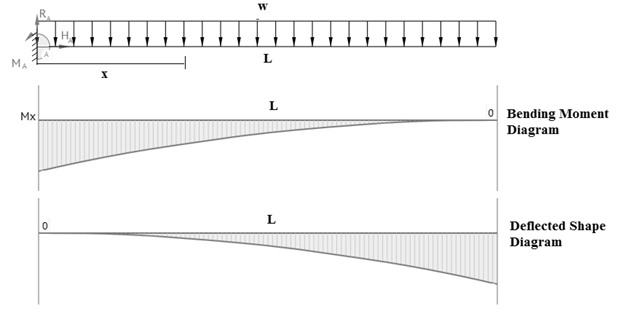Step 2

b) cantilever beam section: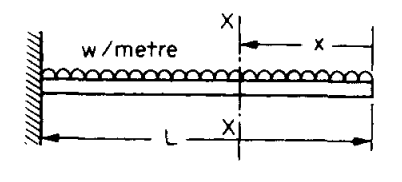Bending moment at section x-x: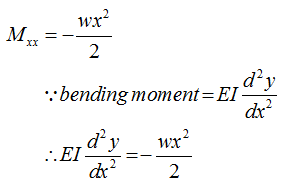Step 3

By doing integration on both sides: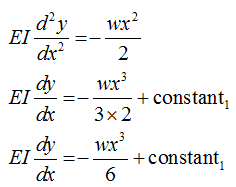Again, do integration on both side: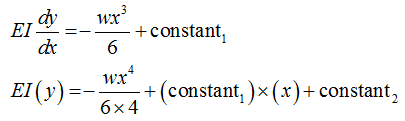...

### Want to see the full answer?

See Solution

#### Want to see this answer and more?

Solutions are written by subject experts who are available 24/7. Questions are typically answered within 1 hour.*

See Solution
*Response times may vary by subject and question.
Tagged in